Simulation of Multistage Countercurrent Liquid-Liquid Extraction

1Department of Chemical Engineering, Pravara Rural Engineering College, Loni, Dist. Ahmednagar (MS), India.

2University Department of Chemical Technology, SGB Amravati University, Amravati, India.

*Corresponding author: Phone: +91 2422 273204; Fax: +91 2422 273537

Abstract

Liquid-Liquid Extraction is one of the most significant unit operations used widely in the chemical industry for the separation of liquid-liquid mixture with the aid of an extracting solvent. There are different contacting patterns for the said purpose viz. co-current, counter current and crosscurrent and also a variety of equipments are encountered. This paper deals with the simulation of counter current liquid-liquid extraction operation for the special case of immiscible solvent using MATLAB. In this paper seven case studies have been studied and the results obtained are compared with the literature and it was found that results are in good agreement with the literature available.

Keywords

Extraction; Counter current; Multistage; Modified McCabe Thiele method; MATLAB

Introduction

Liquid extraction, sometimes called solvent extraction, is the separation of the constituents of a liquid solution by contact with another insoluble liquid. If the substances constituting the original solution distribute themselves differently between the two liquids phases, a certain degree of separation will result, and this can be enhanced by use of multiple contacts or their equivalent in the manner of gas absorption and distillation . In other words Liquid-Liquid extraction refers to a separation of constituents of liquid mixture by contacting it with suitable insoluble liquid solvent, which preferentially dissolves one or more constituents. In liquid extraction, the feed solution to be handled represents one phase and solvent to be used to effect separation, represents the second phase. In this operation, the two immiscible phases in contact are both liquid and so is a liquid-liquid operation. The mass transfer of solute (liquid) takes place from feed solution to the solvent phase . Extraction utilizes differences in the solubilities rather than differences in their volatilities. Since solubilities depend on chemical properties, extraction exploits chemical difference instead of vapour pressure differences. The simplest example involves the transfer of one component from a binary mixture into a second immiscible liquid phase. One example is liquid-liquid extraction of an impurity from wastewater into an organic solvent. This is analogous to stripping or absorption in which mass is transferred from one phase to another. Transfer of the dissolved component (solute) may be enhanced by the addition of “salting out” agents to the feed mixture or by adding “complexing” agents to the extraction solvent. Or in some cases a chemical reaction can be used to enhance the transfer, an example being the use of an aqueous caustic solution to remove phenolics from a hydrocarbon stream. A more sophisticated concept of liquid-liquid fractionation can be used in a process to separate two solutes completely. A primary extraction solvent is used to extract one of the solutes from a mixture (similar to stripping in distillation), and a wash solvent is used to scrub the extract free from the second solute (similar to rectification in distillation).

Separation by distillation becomes expensive, ineffective and difficult when:

¸        Very large amount of latent heats are required (as in case with very dilute solutions where water must be vaporized, latent heats of organic liquids are substantially lower than that of water).

¸        Whenever we are dealing with liquid mixture forming azeotrope / close boiling mixtures.

¸        Whenever we are dealing with substances that are thermally unstable / heat sensitive

In such cases, extraction is attractive and preferred. Some examples are:

¸        Separation of acetic acid from dilute solution with water is done more economically by extraction followed by distillation than distillation alone because of high latent heat of vaporization of water.

¸        Long chain fatty acids can be removed from vegetable oils economically by extraction with liquid propane than high vacuum distillation technique.

¸        Separation of petroleum products that have about same boiling range. Separation of aromatics from lube oil fractions using furfural as a solvent.

¸        Pharmaceutical products that are produced in mixtures which are complex eg. separation of penicillin from fermentation mixtures.

In this paper were explored the possibility of simulation with MATLAB for the special case of immiscible solvent when counter current liquid-liquid extraction were operated. Seven case studies have been simulated and the results obtained are compared with the literature.

Material and Method

Steps Involved

A typical liquid-liquid extraction utilizing the differences in solubilities of components of liquid mixture may be described as consisting of following steps (Fig. 1):

1.      Contacting the feed with a solvent.

2.      Separation of the resulting phases.

3.      Removal / Recovery of solvent(s) from each phase.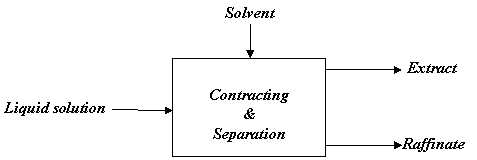Figure 1. Block diagram for liquid extraction

In such extraction operation, the liquid solution and an insoluble solvent are brought into intimate contact, the constituents of liquid mixture are distributed between the phases resulting into some degree of separation (which can be improved by multistage contact), and then phases are separated from one another based on density difference. For example, acetone may be preferentially extracted from a solution in water with the help of chloroform and resulting chloroform phase is found to contain large part of acetone, but little water.

Modified McCabe Thiele Method

The separation achieved for a given number of ideal stages in a counter flow cascade can be determined by using a triangular diagram and special graphical techniques but a modified McCabe Thiele method, which is the approach, used here, is simple to use and has satisfactory accuracy for most cases. The method focuses on the concentration of solute in extract and raffinate phases, and the diagram does not show the concentration of the diluents in the extract or the concentration of solvent in the raffinate. However, these minor components of both phases are accounted for in determining the total flow of extract and raffinate, which affects the position of operating line.

To apply the McCabe Thiele method to extraction, the equilibrium data are shown on graph, where the mass fraction of solute in the extract is plotted on the ordinate and the mass fraction of solute in the raffinate phase as the abscissa. For a type ‘I’ system, the equilibrium line ends with equal compositions at the plait point. The use of only one concentration to characterize a ternary mixture may seem strange, but if the phases leaving a given stage are in equilibrium, only one concentration is needed to fix composition of both phases.

The operating line for the extraction diagram is based on the equation 1: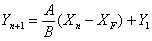(1)

where ‘A’ = Feed Solvent, ‘B’= Extracting Solvent, Xn = Kg. Solute / Kg. Feed solvent in raffinate leaving nth stage, XF = Kg. Solute / Kg Feed solvent in feed entering first stage, Yn+1 = Kg. Solute / Kg. Extracting solvent entering nth stage, Y1 = Kg. Solute / Kg. Extracting solvent leaving first stage.

The Eq.1 gives the relationship between the solute concentration leaving stage n in the raffinate phase and that coming from stage n+1 in the extract phase. The terminal points on the operating line (XF, Y1) and (Xn, Yn+1), are usually determined by an overall material balance, taking into account the ternary equilibrium data. Because of the decrease in the raffinate phase and increase in the extract phase as they pass through the column, the operating line is curved. A material balance over the portion of cascade is made to establish one or more intermediate points on the operating line. The number of ideal stages is then determined by drawing steps in normal manner.

If the number of ideal stages is specified, the fraction of solute extracted and the final compositions are determined by trial and error. The fraction extracted or the final extract composition is assumed, and the curved operating line is constructed. If too many stages are required, a smaller fraction extracted is assumed and the calculations are repeated. Computer programs for extraction calculations are available .

Counter-Current Multistage Extraction

Design procedures and performance analysis of extraction columns are similar to those used for other countercurrent separation processes. Figure 2 shows a schematic diagram of the continuous multistage extraction. It is assumed that the extraction solvent and the feed solution are insoluble and remain so at all concentrations of the distributed solute occurring in the operation. It is also assumed that each stage is an equilibrium stage, and therefore the raffinate and the extract are leaving each stage in equilibrium.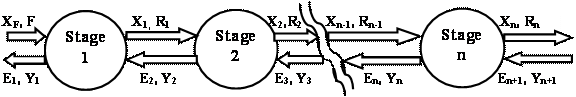Figure 2. Counter-current multistage extraction

Consider that A and B are completely immiscible so that the solvent content of all extracts and the A content of all raffinates are constant (i.e. A kg of A in feed solution will be there in the raffinate leaving each stage of the cascade and the same is true for B kg of B in extract) .

Concentration of solute C is expressed as mass ratio as:

§         X= x / (1-x) = mass C / mass A in raffinate phase

§         Y= y / (1-y) = mass C / mass B in extract phase

§         Ys = mass C / mass B in solvent used

Material balance of the C over stage – 1:

AXF + BY2 = AX1 + BY1                                                                                          I

Material Balance of Solute C over entire unit is:

AXF + BYn+1 = AXn + BY1

A (XF - Xn) = B (Y1 – Yn+1)                                                                                      II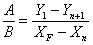III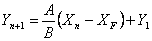IV

where Yn+1 = Ys = mass ratio of solute in solvent used.

Equation (III) is the equation of straight line of slope A / B (on X-Y diagram). This is the operating line which passes through points P (XF, Y1) and Q [Xn, Yn+1 (=Ys)].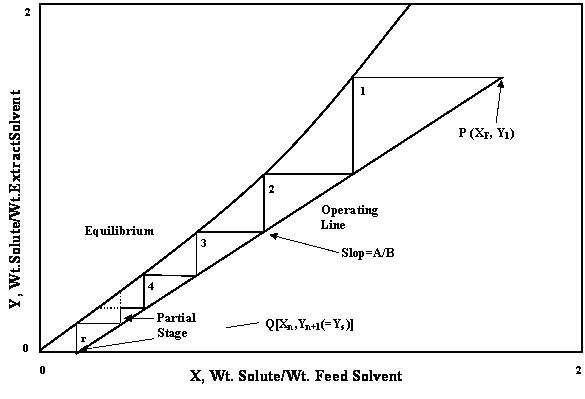Figure 3. Graphical calculation of counter current theoretical stages (for immiscible liquids)

Programming

The computer program using the MATLAB package has been made to find the no. of theoretical stages & other results for the minimum solvent requirement.

Program Output

1. Solute in feed solution (mass/time)

2. Solvent in feed solution (mass/time)

3. Solute in raffinate (mass/time)

4. Solute extracted from feed (mass/time)

5. Minimum solvent requirement for the extraction

6. Number of ideal stages required for the desired separation (N)

Procedure to Calculate Theoretical Stages using McCabe - Thiele Method

Perform an overall material balance to determine terminal compositions & flow rates.

Generating & Plotting the X-Y Equilibrium Data

Plot the X-Y equilibrium data given for the specific case of solute and solvent on the graph with composition of Extracting phase as ordinate and that of Raffinate phase as abscissa. The curve presented in figure 4 is called the equilibrium curve.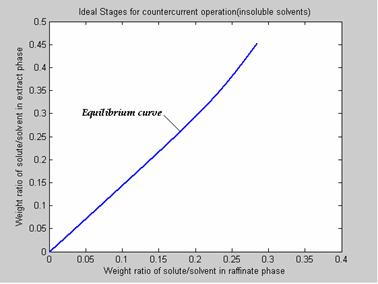Figure 4. Plotting the equilibrium data

Plotting operating line: The operating line is plotted by first locating the points P (XF, Y1) & Q (Xn, Yn+1) in the figure 5. Join points P and Q to get the Operating line.

The slope of this line is given by the ration of A and B i.e. Slope = A / B.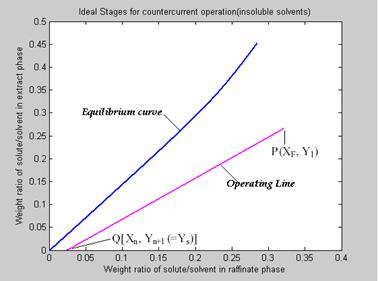Figure 5. Plotting the operating line

Constructing the Theoretical Stages

Now the ideal or theoretical stages are constructed either from top or bottom. Start construction by stepping off stages between operating line and equilibrium curve and continue construction of stages till other end of operating line is reached.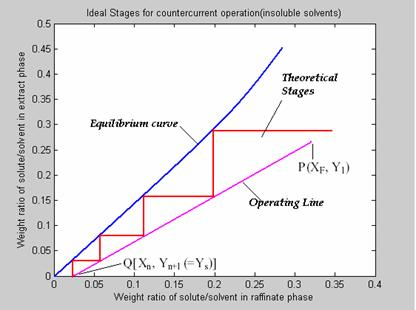Figure 6. Plotting the theoretical stages

Count the number of stages formed. This is the total number of theoretical stages required for complete extraction.

Minimum Solvent Required

Graphical construction for minimum solvent requirement is as shown in figure:

1)      Draw equilibrium curve.

2)      Locate point L (Xn, Yn+1).

3)      Through XF draw vertical to cut the equilibrium curve at K.

4)      Join KL.

5)      The slope of KL is equal to A / Bmin.

6)      With this slope find out Bmin i.e. minimum solvent required.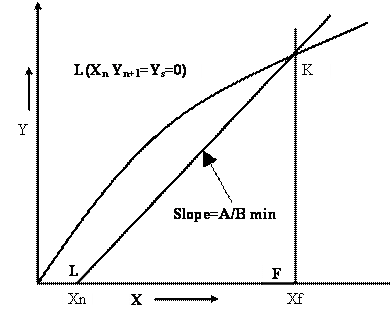Figure 7. Graphical method to find Minimum solvent, counter current extraction

(immiscible solvents)

Results and Discussion

The results and discussions are given in the form of case studies. It was found that the simulated results are in good agreement with available literature.

Case Study 1

If 1000kg / hr of Nicotine (C) – Water (A) solution containing 1% Nicotine is to be counter currently extracted with Kerosene at 20 °C to reduce the Nicotine content to 0.1%, determine: (a) The minimum Kerosene rate and (b) The number of theoretical stages required if 1150 kg of Kerosene is used per hour. The results are presented in figure 7.

Operating Data

X =0    0.0010    0.0025    0.0050    0.0075    0.0100    0.0204

Y =0    0.0008    0.0020    0.0046    0.0069    0.0091    0.0187

Enter the value of Xf (mass solute/total mass in feed): 0.01

Enter the value of Xn (mass solute/total mass in raffinate): 0.001

Enter the feed rate (mass solute/mass solvent in extract phase): 1000

Enter the extracting solvent rate (mass solute/mass solvent in extracting solvent): 1150

Enter the weight fraction of solute in extracting solvent: 0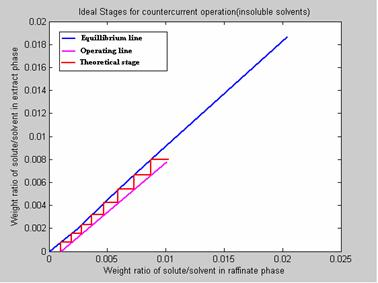Figure 7. Program Results

1. Solute in feed solution (mass/time): 10.000000

2. Solvent in feed solution (mass/time): 990.000000

3. Solute in raffinate (mass/time): 0.990991

4. Solute extracted from feed (mass/time): 9.009009

5. Minimum solvent requirement for the extraction: 974.880820

6. NO. OF IDEAL STAGES REQUIRED FOR THE DESIRED SEPARATION: 8

Case Study 2

A 25% solution of Dioxane in Water is to be continuously extracted at a rate of 1000Kg / hr in counter current extraction system with Benzene to remove 95% of Dioxane. Benzene and Water are substantially insoluble. Determine no. of theoretical stages required if 900 kg of Benzene is used per hour.

Operating Data:

X =0    0.0537    0.2330    0.3369

Y =0    0.0548    0.2903    0.4706

Enter the value of Xf (mass solute/total mass in feed): 0.25

Enter the value of Xn (mass solute/total mass in raffinate): 0.01667

Enter the feed rate (mass solute/mass solvent in extract phase): 1000

Enter the extracting solvent rate (mass solute/mass solvent in extracting solvent): 900

Enter the weight fraction of solute in extracting solvent: 0

Program Results

1. Solute in feed solution (mass/time): 250.000000

2. Solvent in feed solution (mass/time): 750.000000

3. Solute in raffinate (mass/time): 12.714450

4. Solute extracted from feed (mass/time): 237.285550

5. Minimum solvent requirement for the extraction: 510.939043

6. NO. OF IDEAL STAGES REQUIRED FOR THE DESIRED SEPARATION: 6

Case Study 3

An inlet water solution of 100 Kg / hr containing 0.010 wt. fraction Nicotine (A) in Water (C) is stripped with a Kerosene (B) stream of 200 kg /hr containing 0.0005 wt. fraction of Nicotine in a counter current stage tower. The water and Kerosene are essentially immiscible in each other. It is desired to reduce the concentration of the exit water to 0.0010 wt. fraction nicotine. The results are presented in figure 8.

Operating Data:

X = 0    0.0010    0.0025    0.0050    0.0075    0.0099    0.0202

Y = 0    0.0008    0.0020    0.0045    0.0068    0.0090    0.0185

Enter the value of Xf (mass solute/total mass in feed): 0.010

Enter the value of Xn (mass solute/total mass in raffinate): 0.0010

Enter the feed rate (mass solute/mass solvent in extract phase): 100

Enter the extracting solvent rate (mass solute/mass solvent in extracting solvent): 200

Enter the weight fraction of solute in extracting solvent: 0.0005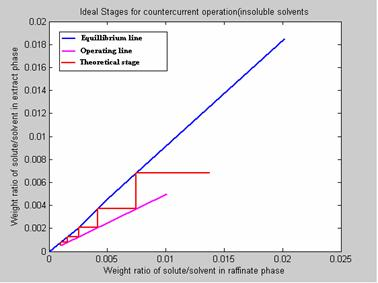Figure 8. Program Results

1. Solute in feed solution (mass/time): 1.000000

2. Solvent in feed solution (mass/time): 99.000000

3. Solute in raffinate (mass/time): 0.099099

4. Solute extracted from feed (mass/time): 0.900901

5. Minimum solvent requirement for the extraction: 97.472748

6. NO. OF IDEAL STAGES REQUIRED FOR THE DESIRED SEPARATION: 5

Case Study 4

If 8000 kg/hr of an Acetic acid (C) – Water (A) solution containing 30% acid, is to be counter currently extracted with Isopropyl Ether (B) to reduce the acid concentration to 2% in the solvent free raffinate product, determine: (a) Minimum amount of solvent which can be used and (b) No. of theoretical stages if 20,000 Kg / hr of solvent is used.

Operating Data

X = 0    0.0069    0.0143    0.0298    0.0686    0.1534    0.3423    0.5798    0.7953    0.8647

Y = 0    0.0018    0.0037    0.0080    0.0197    0.0506    0.1287    0.2754    0.4514    0.5674

Enter the value of Xf (mass solute/total mass in feed): 0.30

Enter the value of Xn (mass solute/total mass in raffinate): 0.02

Enter the feed rate (mass solute/mass solvent in extract phase): 8000

Enter the extracting solvent rate (mass solute/mass solvent in extracting solvent): 20000

Enter the weight fraction of solute in extracting solvent: 0

Program Results

1. Solute in feed solution (mass/time): 2400.000000

2. Solvent in feed solution (mass/time): 5600.000000

3. Solute in raffinate (mass/time): 114.285714

4. Solute extracted from feed (mass/time): 2285.714286

5. Minimum solvent requirement for the extraction: 12560.568400

6. NO. OF IDEAL STAGES REQUIRED FOR THE DESIRED SEPARATION: 9

Case Study 5

A counter current extraction plant is used to extract Acetone (A) from its mixture with water by means of Methyl Isobutyl Ketone (MIK) at a temp. of 25 °C. The feed consists of 40 % Acetone and 60 % Water. Pure solvent equal in mass to the feed is used as the extracting liquid. How many ideal stages are required to extract 99% of Acetone fed? What is the extract composition after the removal of solvent?

Operating Data:

X = 0    0.0250    0.0550    0.0750    0.1000    0.1250    0.1550    0.1750    0.2000    0.2250    0.2500    0.2600

Y = 0    0.0450    0.1000    0.1350    0.1750    0.2130    0.2550    0.2820    0.3120    0.3400    0.3650    0.3750

Enter the value of Xf (mass solute/total mass in feed): 0.40

Enter the value of Xn (mass solute/total mass in raffinate): 0.0067

Enter the feed rate (mass solute/mass solvent in extract phase): 100

Enter the extracting solvent rate (mass solute/mass solvent in extracting solvent): 100

Enter the weight fraction of solute in extracting solvent: 0

Program Results

1. Solute in feed solution (mass/time): 40.000000

2. Solvent in feed solution (mass/time): 60.000000

3. Solute in raffinate (mass/time): 0.404712

4. Solute extracted from feed (mass/time): 39.595288

5. Minimum solvent requirement for the extraction: NaN

6. NO. OF IDEAL STAGES REQUIRED FOR THE DESIRED SEPARATION: 4

Case Study 6

100 Kg/hr of a Nicotine-Water containing 0.01 wt. fraction Nicotine is extracted with 150 kg/hr of Kerosene containing 0.0006 wt. fraction Nicotine in counter current stage column. Nicotine content of exit water stream is 0.0010 wt. fraction. Determine the no. of theoretical stages required for the separation. Water and Kerosene are extensively immiscible solvents. The results are presented in figure 9.

Operating Data:

X =         0    0.0010    0.0025    0.0050    0.0075    0.0100    0.0204

Y =         0    0.0008    0.0020    0.0046    0.0069    0.0091    0.0187

Enter the value of Xf (mass solute/total mass in feed): 0.01

Enter the value of Xn (mass solute/total mass in raffinate): 0.0010

Enter the feed rate (mass solute/mass solvent in extract phase): 100

Enter the extracting solvent rate (mass solute/mass solvent in extracting solvent): 150

Enter the weight fraction of solute in extracting solvent: 0.0006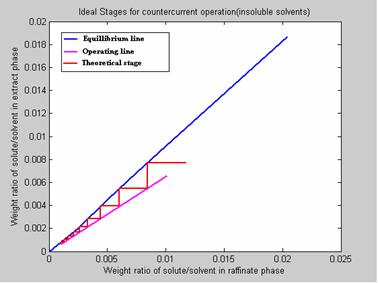Figure 9. Program Results

1. Solute in feed solution (mass/time): 1.000000

2. Solvent in feed solution (mass/time): 99.000000

3. Solute in raffinate (mass/time): 0.099099

4. Solute extracted from feed (mass/time): 0.900901

5. Minimum solvent requirement for the extraction: 97.488082

6. NO. OF IDEAL STAGES REQUIRED FOR THE DESIRED SEPARATION: 9

Case Study 7

A 100-kg/h feed stream containing 20wt. % Acetic acid in Water is to be extracted with 200 kg/h of recycle MIK that contains 0.1 percent acetic acid and 0.01 percent water. The aqueous raffinate is to be extracted down to 1 percent acetic acid. How many theoretical stages will be required and what will the extract composition be? The results are presented in figure 10.

Operating data:

X = 0    0.0299    0.1364    0.2708    0.3864    0.5964    0.8065

Y = 0    0.0196    0.1039    0.2354    0.4039    0.6525    0.9492

Enter the value of Xf (mass solute/total mass in feed): 0.20

Enter the value of Xn (mass solute/total mass in raffinate): 0.01

Enter the feed rate (mass solute/mass solvent in extract phase): 100

Enter the extracting solvent rate (mass solute/mass solvent in extracting solvent): 200

Enter the weight fraction of solute in extracting solvent: 0.001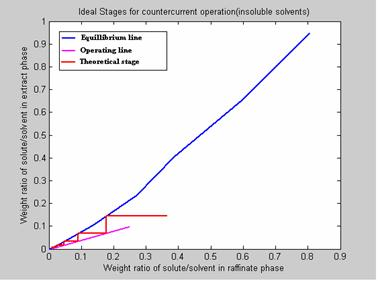Figure 10. Program Results

1. Solute in feed solution (mass/time): 20.000000

2. Solvent in feed solution (mass/time): 80.000000

3. Solute in raffinate (mass/time): 0.808081

4. Solute extracted from feed (mass/time): 19.191919

5. Minimum solvent requirement for the extraction: 89.244480

6. NO. OF IDEAL STAGES REQUIRED FOR THE DESIRED SEPARATION: 5

Conclusion

The simulation results have been compared with standard literature available in chemical engineering and it is seen that the results are absolutely identical. So the computer program which is prepared is perfect and can be utilized by students as well as designers for the calculations of the related system and the same program can also be adopted for any extension.

References

1.      Perry R.H., D.W. Green, Perry’s Chemical Engineers’ Handbook, 7th ed., McGraw-Hill, New York, 1997.

2.      Treybal R.E. Mass Transfer Operations, 3rd ed., McGraw-Hill, New York, 1980.

3.      McCabe W.L., Smith J.C., Harriott P. Unit Operations of Chemical Engineering, 6th ed., McGraw-Hill, 2000.

4.      Coulson J.M., Richardson, J.F. Chemical Engineering, Vol. 2, 4th ed. Pergamon Press, 1980.

5.      Roy, G.K. Fundamentals of Heat and Mass Transfer, 5th ed., Khanna Publishers, Delhi, 2004.

6.      Gavhane K.A. Mass Transfer – II, 2nd ed., Nirali Prakashan, Pune, 2004.

7.      Geankoplis C.J. Transport Processes and Unit Operations, 3rd ed., Prentice Hall of India, 2002.